# Incorrect Bboxes

While the masks seem to be correct, it seems that many of the images in the train dataset have bboxes that do not match the masks. While some of the bboxes are merely slightly off, many are drastically off, as we can see in the examples below.

If you are using the bboxes in training, this may cause problems as the model will be attempting to learn using incorrect bboxes. I wrote the following code to recreate the bboxes based on the masks :

``````import json
from pycocotools.coco import COCO

def create_new_bboxes(item, coco_ds):
try:
# convert the item to a binary mask
bin_mask = coco_ds.annToMask(item)

# sum the rows and cols
row_sums = bin_mask.sum(axis=1)
col_sums = bin_mask.sum(axis=0)

# find the first non-zero row
for ty, row in enumerate(row_sums):
if row > 0:
break

# find the first non-zero col
for tx, col in enumerate(col_sums):
if col > 0:
break

# find the first non-zero row from the end
for by in range(len(row_sums) - 1, 0, -1):
if row_sums[by] > 0:
break

# find the first non-zero col from the end
for bx in range(len(col_sums) - 1, 0, -1):
if col_sums[bx] > 0:
break

item['bbox'] = [tx, ty, bx-tx, by-ty]
except Exception as e:
print("Error with image", item['image_id'])
print(e)
return item

def rebbox_dataset(annotations):
# create our coco object
coco_ds = COCO(annotations)

# load the data
with open(annotations) as f:
data = json.loads(f.read())

for i, item in enumerate(data['annotations']):
data[i] = create_new_bboxes(item, coco_ds)

return data
``````

In the images below, the red box is the bbox from the annotation and the blue bbox is a box derived from the mask.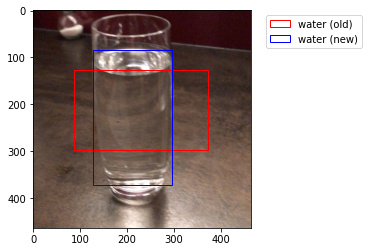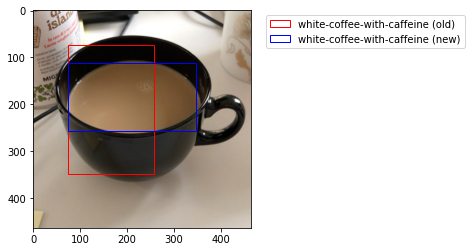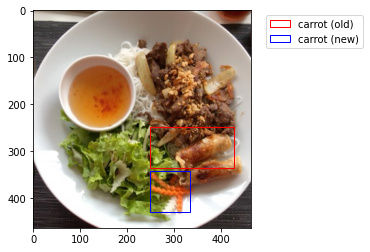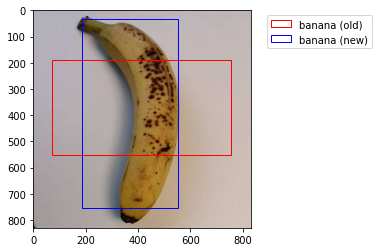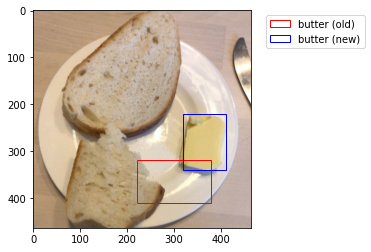2 Likes